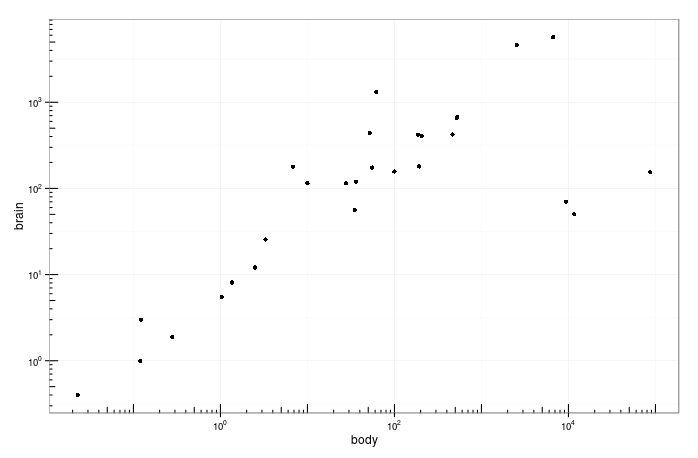## ggplot包不常见函数集合

2013年04月12日

1. scale_fill_continuous 函数有一个limits参数文档中没有介绍，可以用来指定颜色对应数值的范围。类似的scale_xxx_continuous函数将直接删除范围以外的数据点。在不删除数据点的情况下限制坐标轴范围，可以使用coord_cartesian(xlim=c(min, max))等函数。

2. 利用stat_function绘制曲线并利用scale_color_manual手动设置颜色

 ggplot(data.frame(x=c(0,2*pi)),aes(x))+
stat_function(aes(color='sin'),fun=sin)+
stat_function(aes(color='cos'),fun=cos)+
scale_color_manual(values=c('sin'='red',
'cos'='black'))+
labs(color='')

3. 类似guides(color=FALSE)这样可以关闭掉图例。

4. annotation_logticks

 library(ggplot2)
library(MASS)
library(scales)
a <- ggplot(Animals, aes(x = body, y = brain)) + geom_point() +
scale_x_log10(breaks = trans_breaks("log10", function(x) 10^x),
labels = trans_format("log10", math_format(10^.x))) +
scale_y_log10(breaks = trans_breaks("log10", function(x) 10^x),
labels = trans_format("log10", math_format(10^.x))) +
theme_bw()
# 上面使用trans_breaks及trans_format来制作一个函数实现将刻度转化为更好看的形式，而非普通的很多0的数值

a + annotation_logticks()                # Default: log ticks 在下面和左边
a + annotation_logticks(sides = "lr")    # left and right: 在左边和右边（y轴上）
a + annotation_logticks(sides = "trbl")  # All four sides: 四周都有5. 函数adjustcolor(col,alpha)可以将某种颜色转换为具有指定透明度alpha的颜色。一些色板函数如heat.colors等也可以设置alpha参数。包RColorBrewer提供了额外的色板，使用display.brewer.all查看其提供的色板，使用brewer.pal设置色板。

6. 函数facet_grid有一个参数labeller可以用来指定处理子图标题的转换函数。例如采用A~B进行分面，指定函数function(var,value){paste(ifelse(var=='A','A','B'),value,sep=": ")}(v1.0)或function(var){v <- list(paste(ifelse(colnames(var)=='A','A','B'),var[,1])); names(v) <- colnames(var); v}(v2.0)即可在子图标题上添加对应变量名称。v2.0提供了一些函数来快速生成需要的标签函数，比如facet_wrap('alpha',labeller = label_bquote(alpha==.(alpha)))可方便地生成数学表达式。

7. 整个ggplot对象只能使用一个coord_xx函数,因为对坐标轴的变换是在最后一次完成的。所以coord_flipcoord_fixd/coord_equal不能同时使用，要调节图片长款比例可以使用theme(aspect.ratio = 1)

8. scale_*_**等系列函数有expand参数可以调节边界数据点与坐标轴之间的空袭。

9. annotate_custom(grob=ggplotGrob(another.ggplot), xmin=0,xmax=1,ymin=0,ymax=1)可以在指定位置嵌入一个子图。

10. gtablegridExtra包提供了操纵ggplot对象的低级函数，采用如下方式可以将多幅子图(存放在list中比较方便)合并并对齐坐标轴:

rbind.ggplot.list <- function(gl, size='first'){
gl <- lapply(gl, ggplotGrob)
p <- do.call(rbind,args = c(gl,size=size))
p$widths <- do.call(unit.pmax,lapply(pl, function(x){x$widths}))
return(p)
}
pl <- rbind.ggplot.list(pl)
grid.newpage()
grid.draw(pl)

11. 可将多个复用的layer存储在list中，然后一次性加到ggplot对象中。